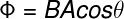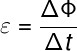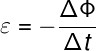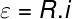Electricity & Megnetism

Faraday’s law , also known as the law of electromagnetic induction , states that a change in the flux of a magnetic field through conductive materials induces an electric current to arise.

The phenomenon of electromagnetic induction was discovered by the British physicist and chemist Michael Faraday in 1831. This discovery was one of the most important in all of history, since, thanks to this phenomenon, we are able to generate energy in hydroelectric plants , produce movement using electric motors , generate heat through induction furnaces make magnetic readings and recordings , and others.

To understand how Faraday’s law works, it is essential that we know the concept of magnetic flux .

## magnetic flux

The magnetic flux (Φ) is a scalar quantity that measures the number of magnetic field lines ( B ) that cross a closed area (A) . In addition, the magnetic flux depends on the angle formed between the magnetic field and the normal line ( N ) in area A. Check the formula used to calculate this flux:Φ – magnetic flux (Wb – weber or T.m²)

B – magnetic field (T – tesla)

A – area (m²)

The following figure illustrates the magnetic flux through a surface of area A, note

Formally, the law of electromagnetic induction is known as the Faraday-Neumann-Lenz law , however it is often just referred to the Faraday’s law . This is because the phenomenon of induction was discovered by Michael Faraday, but its mathematical formulation was made by Franz Ernst Neumann. Furthermore, Heinrich Lenz proposed the need to use the negative sign of the formula, which concerns the direction of the induced electric current.

Faraday’s law was of paramount importance for the development of electromagnetism , since it reveals the existence of a direct relationship between electrical and magnetic phenomena , which were treated as phenomena of a different nature for many years.

## Induced electromotive force

According to Faraday’s law, the emergence of electric currents depends on the change in magnetic flux, so we write that the time variation of the magnetic flux is equivalent to an electric potential measured in volts (V), which, for historical reasons, is called the induced electromotive force (ε).ε – induced electromotive force (V – volts)

ΔΦ = Φ F – Φ i – variation of magnetic flux (Wb)

Δt – time interval (s)

Also, due to the principle of conservation of energy , it is necessary that we add a negative sign to Faraday’s law. This signal was introduced by Lenz’s Law , which allows us to know the direction of the induced electric current:

The union of this information gives rise to the Faraday-Lenz law , check it out:By applying Ohm’s 1st law , it is possible to calculate the electrical resistance or the electrical current that forms in ohmic conductors, thanks to the phenomenon of electromagnetic induction:R – electrical resistance (Ω)

i – electric current (A)

• ### electric motor

In addition to enabling the emergence of energy generators, such as those used in basically all types of power plants , the phenomenon of electromagnetic induction, described by Faraday’s law, allowed us to create the first electric motor.

The electric motor and generators work on the same principle. Inside these motors, there is a conducting loop, arranged between the opposite poles of a configuration of magnets. When an electric current passes through the conducting loop, the magnetic field of the magnets produces a torque on it, causing it to rotate.

• ### transformers

Transformers aredevices used to lower or raise electrical voltages and currents . They consist of two coils: primary and secondary. These are wrapped around a closed iron bar, or arranged in a U-shape.

When an alternating electric current passes through the primary winding, an oscillating magnetic field is produced and guided through the inside of the iron bar, in this way, an electric current is induced in the secondary winding.

The ratio between the number of turns in the primary and secondary windings is what determines the increase or decrease of the input and output electrical voltages.

In 1864, James Clerk Maxwell unified electrical and magnetic phenomena through four equations of electromagnetism , they are: Gauss’s law, Gauss ‘s law for magnetism, Ampère’s law and Faraday’s law . According to Maxwell’s contributions to Faraday’s law , changing magnetic fields produce rotating electric fields and tangent to magnetic field lines.

## Solved exercises on Faraday’s law

Question 1) Regarding the phenomenon of electromagnetic induction, mark the incorrect alternative.

a) When we approach or move a magnet away from a conducting coil, we induce the appearance of an electric current.

b) The induced electromotive force is the name given to the electric potential that is produced by electromagnetic induction.

c) The flux of a magnetic field through a loop induces the appearance of an electric current.

d) The variation of the magnetic field flux induces the formation of electric currents.

e) The induced electric current is proportional to the variation of the magnetic flux.

Template: Letter C

Resolution :

The statement made in letter C is wrong. In reality, the appearance of an induced electric current depends on the variation of the magnetic flux. If the magnetic flux is constant, there will be no production of electric current through electromagnetic induction.

Question 2) The magnetic flux through a conducting loop was 20.0 Wb/s. After a time interval of 2.0 s, this flux becomes zero due to the rotation of the plane of the loop. The magnitude of the electromotive force induced during this rotation was

a) 10.0 V

b) 15.0 V

c) 20.0 V

d) 40.0 V

e) 35.0 V

Template: Letter A

Resolution :

We will calculate the induced electromotive force using the Faraday-Lenz law, note:

Using the data provided in the exercise statement, we find an induced voltage of 10.0 V, so the correct alternative is letter A.

Question 3) Mark the alternative that presents only devices that work through the phenomenon of electromagnetic induction.

a) Transformers, induction ovens, electric pots.

b) Generators, iron, electric shower.

c) Television, radio, incandescent light bulb.

d) Transformers, metal detectors, electric motors.

e) Hair dryer, vacuum cleaner, sandwich maker.

Template: Letter D

Resolution:

Devices that work by means of electromagnetic induction are those that have electric motors or those that make use of oscillating magnetic fields to induce the emergence of electrical voltages. These devices are: transformers, induction furnaces, generators, metal detectors, hair dryers and vacuum cleaners, for example. Therefore, the correct alternative is letter D.